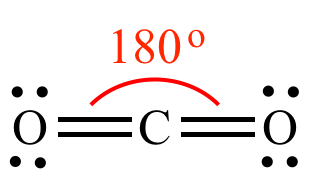Illustrated Glossary of Organic Chemistry

VSEPR Theory: Valence Shell Electron Pair Repulsion Theory. A theory to explain and predict molecular structure, based on lone pairs (nonbonded electron pairs), and in electron pairs in bonds. It is assumed that the molecule adopts a geometry that moves the electron pairs as far apart as possible in order to minimize electrostatic repulsion. The number of electron groups around the central atom is sometimes called the steric number. VSEPR theory is intended to give only an initial guess at molecular structure. Basic VSEPR theory is not intended to be quantitative, and cannot be used to predict precise bond angles. An exception is when all bond angles are equal (square planar, tetrahedral, etc.) or otherwise easily predicted, in which case the bond angles can be calculated using basic geometry.VSEPR theory predicts a linear structure for carbon dioxide (instead of a bent structure) because a linear structure places the four bonding electrons pairs (two pairs in each double bond) as far apart as possible.VSEPR theory predicts methane is a perfect tetrahedron with all H-C-H bond angles equal at 109.5o, because the hydrogen atoms repel equally, and because this geometry puts the greatest distance between all four bonded electrons pairs.VSEPR theory predicts ammonia is distorted tetrahedron (a pyramid), because this geometry puts the greatest distance between the three bonded electron pairs and the lone pair. A lone pair occupies more space (i.e., has stronger repulsion) than a hydrogen atom, so the lone pair-N-H bond angles are greater than the perfect 109.5o tetrahedral bond angles found in methane, and the H-N-H bond angles are compressed to less than 109.5o. (The empirical H-N-H bond angles in ammonia are 107.8o.)